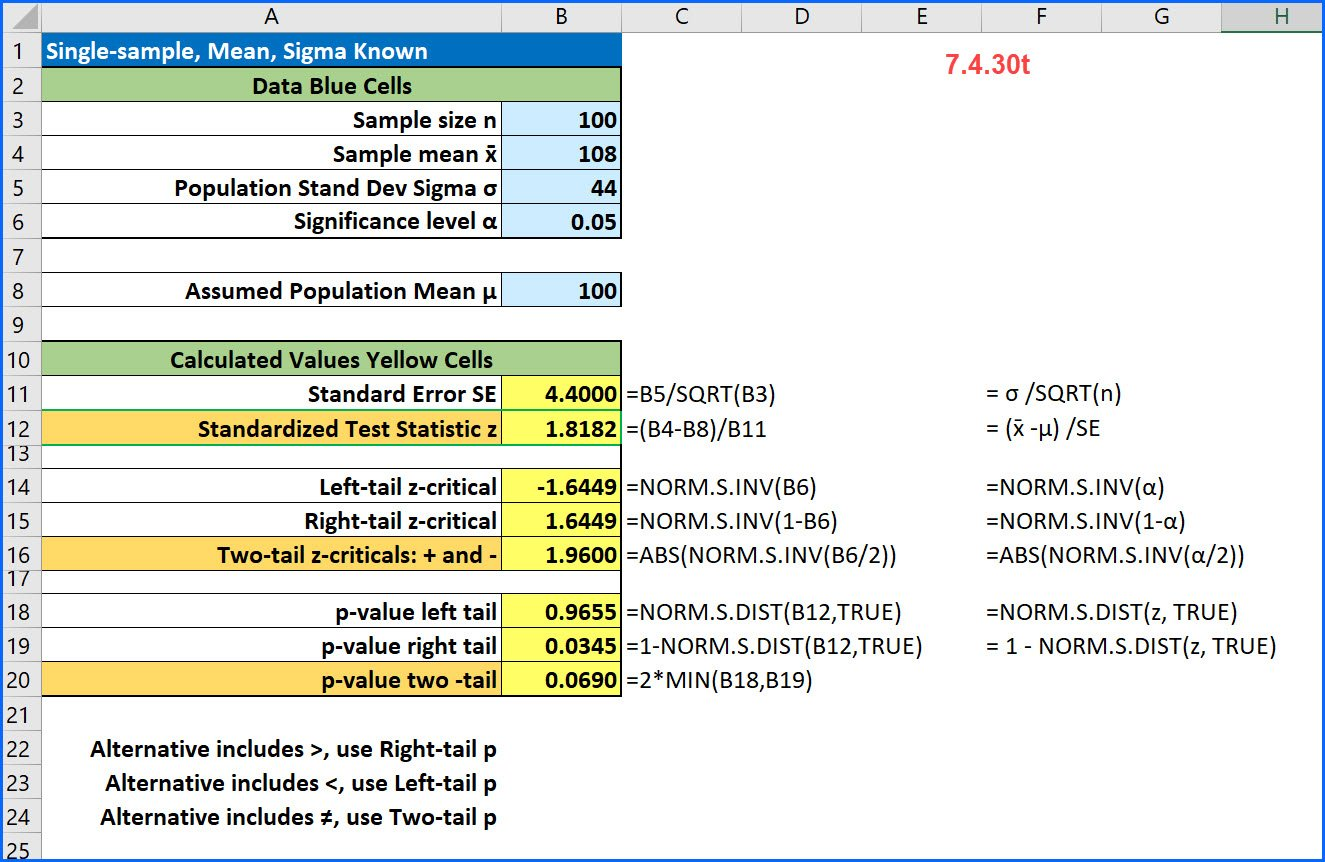# BUS 233## Finite Population Correction factor

The Finite Population Correction Factor, sometimes just called the FPC factor, is used when the sample size is large relative to the population size. For most situations, the population is so large, typical sample sizes are far too small to worry about the need for the FPC. The guidance is that we need to use …## Single-sample z-test for the mean [7.4.30t]

Here is a common problem from intro stats: [7.4.30t] A random sample of 100 observations from a population with a standard deviation of 44 yielded a sample mean of 108. Test the null hypothesis that μ = 100 against the alternative that μ > 100 at an alpha of 0.05. Here because the alternative contains …

## 8.4.14 Difference Between Proportions StatCrunch

A Two-sample problem for testing to see if the two proportions are different:

## One-sample Sigma Known z-test for the Mean

Joel, I have not checked all your calculations, but the process you used looks good. I note that this is a problem where you are given a lot of raw data. Which technology did you use? I ask because problems where you have to manually enter a lot of raw data often lead to mistakes …

## How to State the Conclusion about a Hypothesis Test

After you have completed the statistical analysis and decided to reject or fail to reject the Null hypothesis, you need to state your conclusion about the claim. To get the correct wording, you need to recall which hypothesis was the claim. If the claim was the null, then your conclusion is about whether there was …